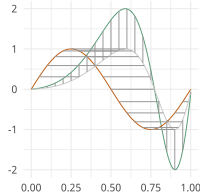# UMAP EFDA Metrics¶

Distance metrics for functions and curves in R^n for use with UMAP (https://github.com/lmcinnes/umap)

moduleauthor:: J. Derek Tucker <jdtuck@sandia.gov>

umap_metric.efda_distance[source]

” calculates the distances between two curves, where q2 is aligned to q1. In other words calculates the elastic distances/ This metric is set up for use with UMAP or t-sne from scikit-learn

Parameters: q1 – vector of size N q2 – vector of size N scalar amplitude distance
umap_metric.efda_distance_curve[source]

” calculates the distances between two curves, where beta2 is aligned to beta1. In other words calculates the elastic distance. This metric is set up for use with UMAP or t-sne from scikit-learn

Parameters: beta1 – vector of size n*M beta2 – vector of size n*M closed – if open curves and (1) if closed curves scalar shape distance